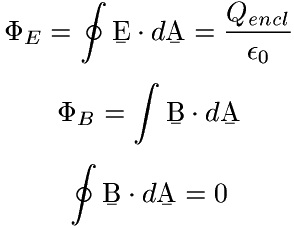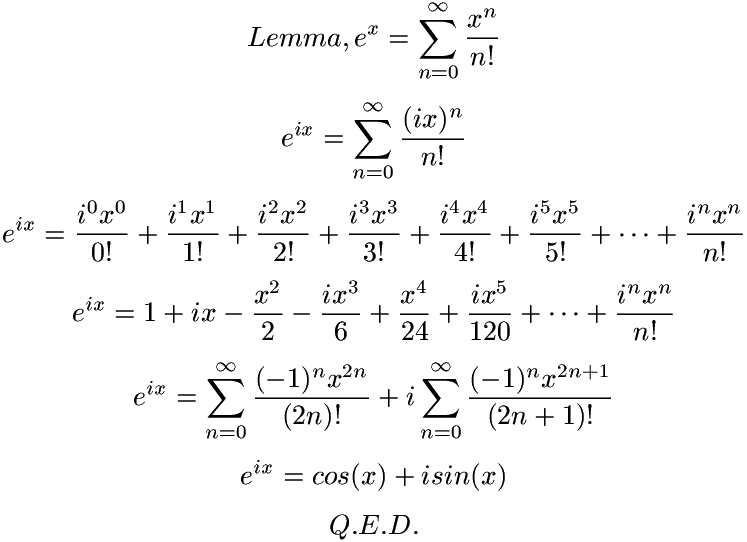Converting TeX/LaTeX to PNG You don't need me to tell you that TeX and LaTeX are awesome. However, sometimes having a TeX or LaTeX document in DVI, PDF, etc. is cumbersome. There are other times when latex2html is overkill. Sometimes you just want to make a formula into a PNG image (or JPG or whatever) instead of a report. This page explains how to do that. Before you continue, you will need the following: A working TeX/LaTeX installation texi2dvi dvips ImageMagick A sample TeX/LaTeX file A sample file is available here. The first thing you'll want to do is make sure your TeX/LaTeX installation and ImageMagick installation work. Determining this is beyond the scope of this document. Please look elsewhere if you need assistance (Tug.org might be a good idea). Once you have a working installation, there are a few things that need to be put in the TeX/LaTeX file to make it look nice. Here are the contents of the sample file:  \documentclass{article} \pagestyle{empty} \begin{document} $$\Phi_E = \oint \bmath{E} \cdot d\bmath{A} = \frac{Q_{encl}}{\epsilon_0}$$ $$\Phi_B = \int \bmath{B} \cdot d\bmath{A}$$ $$\oint \bmath{B} \cdot d\bmath{A} = 0$$ \end{document}  Most of this should look framiliar. The only thing that may stand out (besides the formulae) is the \pagestyle{empty}. All this does is turn off the page number. You'll want to do this because if you don't, you'll have one huge PNG image with just the formula and tons of whitespace below it and the page number on the bottom. Since you probably don't want to do this, it's a good idea to turn the page numbers off. Now it's time to use the command line.  # texi2dvi flux.tex # dvips -E flux.dvi # convert -density 200x200 flux.ps flux.png  The first thing we do is create a DVI from the TeX document. Then we convert the dvi into encapsulated postscript. Finally, we convert the postscript into a PNG using ImageMagick. The density option in the convert command changes the size of the image. Almost all of the images on this site use the 200x200. I have found that 90x90 seems to be about "normal size". If you don't want to use PNG, just change the extension, as long as it's supported by ImageMagick it should work. Here's the image that was generated by these commands (looks like I don't have any bold math fonts installed).Of course, if you have the ambition, you can make more elaborate images too, like this:This is technically not a proof though since Euler's formula is actually a definition (from what I am told). At any rate, I hope that this method worked for you and that it will help you in the future.